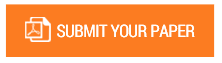Determination of Risk Types of Price, Lumpsum and Target Price Contract (THB) Using Analytic Hierarchi Process (AHP) Method

Authors : Niken Empina Putri; Erry Rimawan; Erik Odi Wijaya; Setiawan; Ojakma Sihar P.T

Volume/Issue : Volume 5 - 2020, Issue 12 - December

Scribd : https://bit.ly/38uQAWt

In a project that uses a lump sum as a reference in submitting a bid is a design drawing so that high accuracy is needed in reading the image and calculating the Bill of Quantity (BOQ) and the Budget Plan (RAB). With the THB contract, the owner and contractor agree with their best efforts to review the design including the selection of materials and equipment in order to achieve the optimal contract value. In this case, the risk that is experienced is that it takes a long time to calculate progress so that it can disrupt cash flow which results in delays in implementation. The purpose of this study is to identify and determine the factors that affect the project risk from the unit price contract type, lump sum and wholesale price target (THB). Determine the type of contract that has the least risk in choosing the type of contract. From the factor weights that have been calculated using the Analytic Hierarchy Process (AHP) method, it is explained that the lump sum contract type is indeed relatively more risky to the ratio of profit to risk in project implementation. Whereas the type of contract with the least risk is the wholesale price target with the score of each type of contract, the unit price is in the second rank with a score of 0.423, lump sum is in the third rank with a score of 0.418, and THB is in the first position with a score of 0.469. So that the implementation is more efficient using the Contract Price Target (THB) type. From the factor weights that have been calculated using the Analytic Hierarchy Process (AHP) method, it is explained that the lump sum contract type is indeed relatively more risky to the ratio of profit to risk in project implementation. While the type of contract with the least risk is the wholesale price target with a score for each type of contract, the unit price is in the second rank with a score of 0.423, lump sum is in the third rank with a score of 0.418, and THB is in the first position with a score of 0.469. So that the implementation is more efficient using the Contract Price Target (THB) type. From the factor weights that have been calculated using the Analytic Hierarchy Process (AHP) method, it is explained that the lump sum contract type is indeed relatively more risky to the ratio of profit to risk in project implementation. While the type of contract with the least risk is the wholesale price target with a score for each type of contract, the unit price is in the second rank with a score of 0.423, lump sum is in the third rank with a score of 0.418, and THB is in the first position with a score of 0.469. So that the implementation is more efficient using the Contract Price Target (THB) type. While the type of contract with the least risk is the wholesale price target with a score for each type of contract, the unit price is in the second rank with a score of 0.423, lump sum is in the third rank with a score of 0.418, and THB is in the first position with a score of 0.469. So that the implementation is more efficient using the Contract Price Target (THB) type. While the type of contract with the least risk is the wholesale price target with a score for each type of contract, the unit price is in the second rank with a score of 0.423, lump sum is in the third rank with a score of 0.418, and THB is in the first position with a score of 0.469. So that the implementation is more efficient using the Contract Price Target (THB) type

Keywords : Lump Sum, Unit Price, THB, Risk, AHP.

#### CALL FOR PAPERS

Paper Submission Last Date
31 - May - 2022